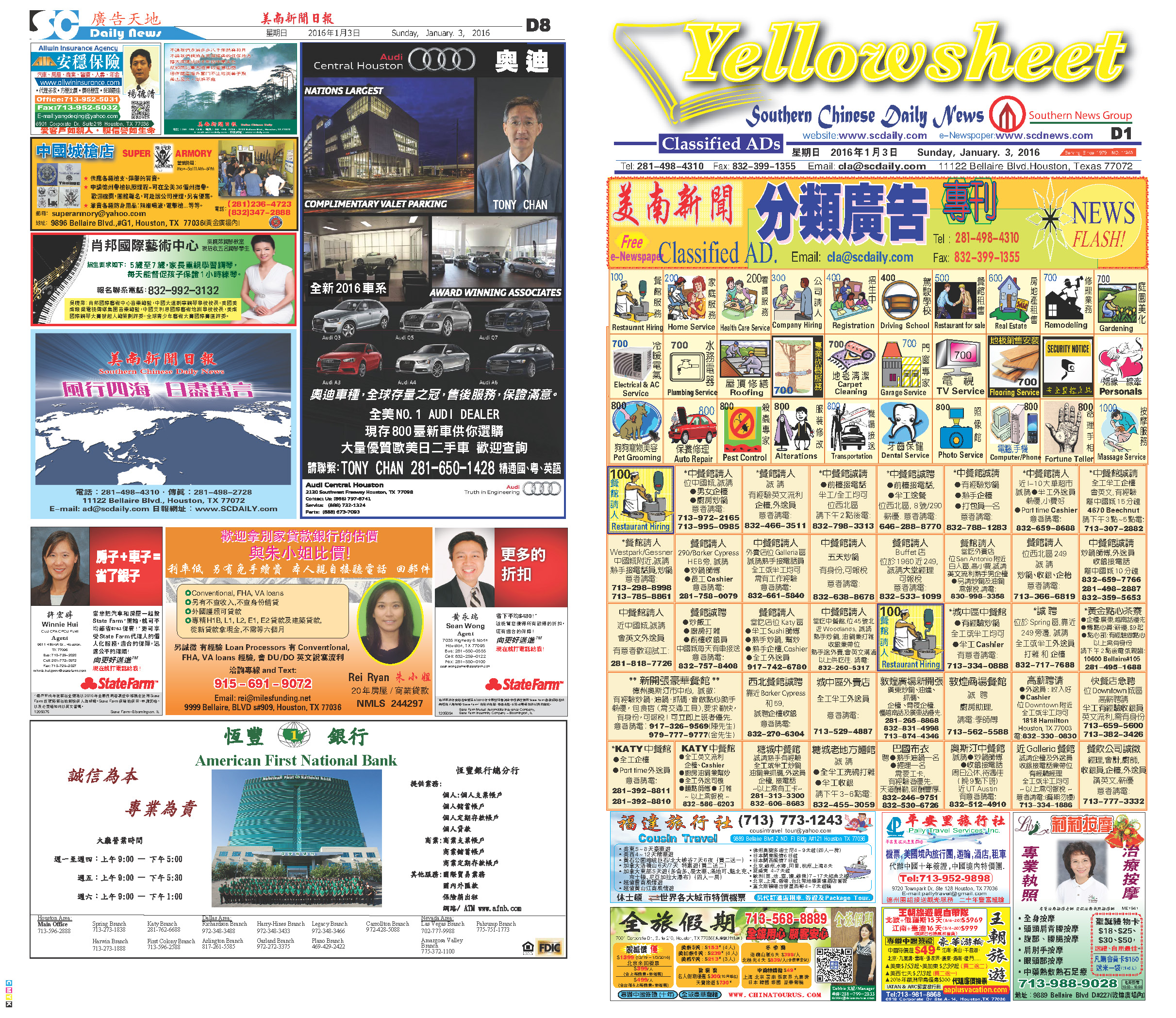160113 Epaper

 A Section B SectionC Section D Section160112 Epaper

 A Section B SectionC Section D Section160111 Epaper

 A Section B SectionC Section D Section160110 Epaper

 A Section B Section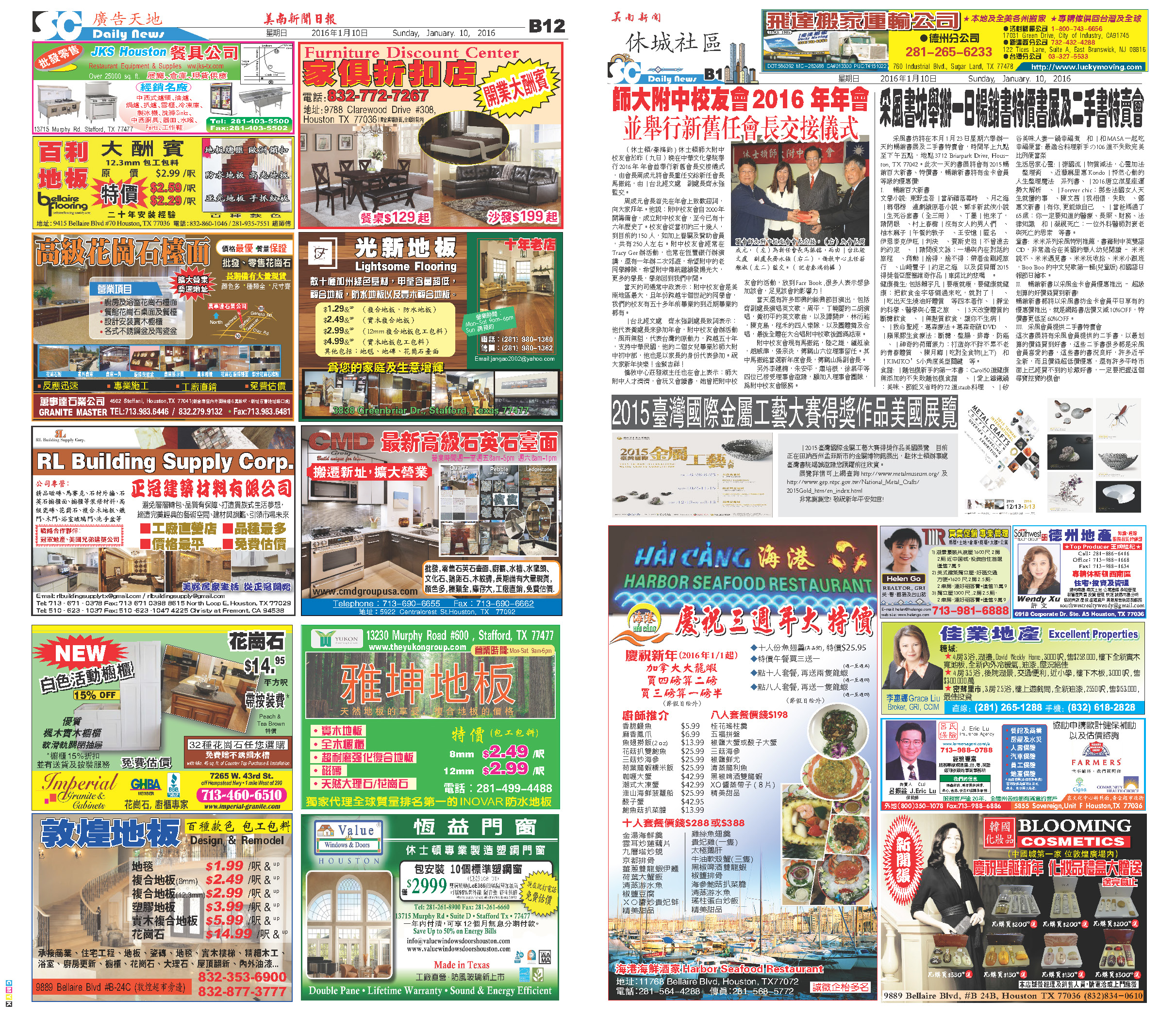C Section D Section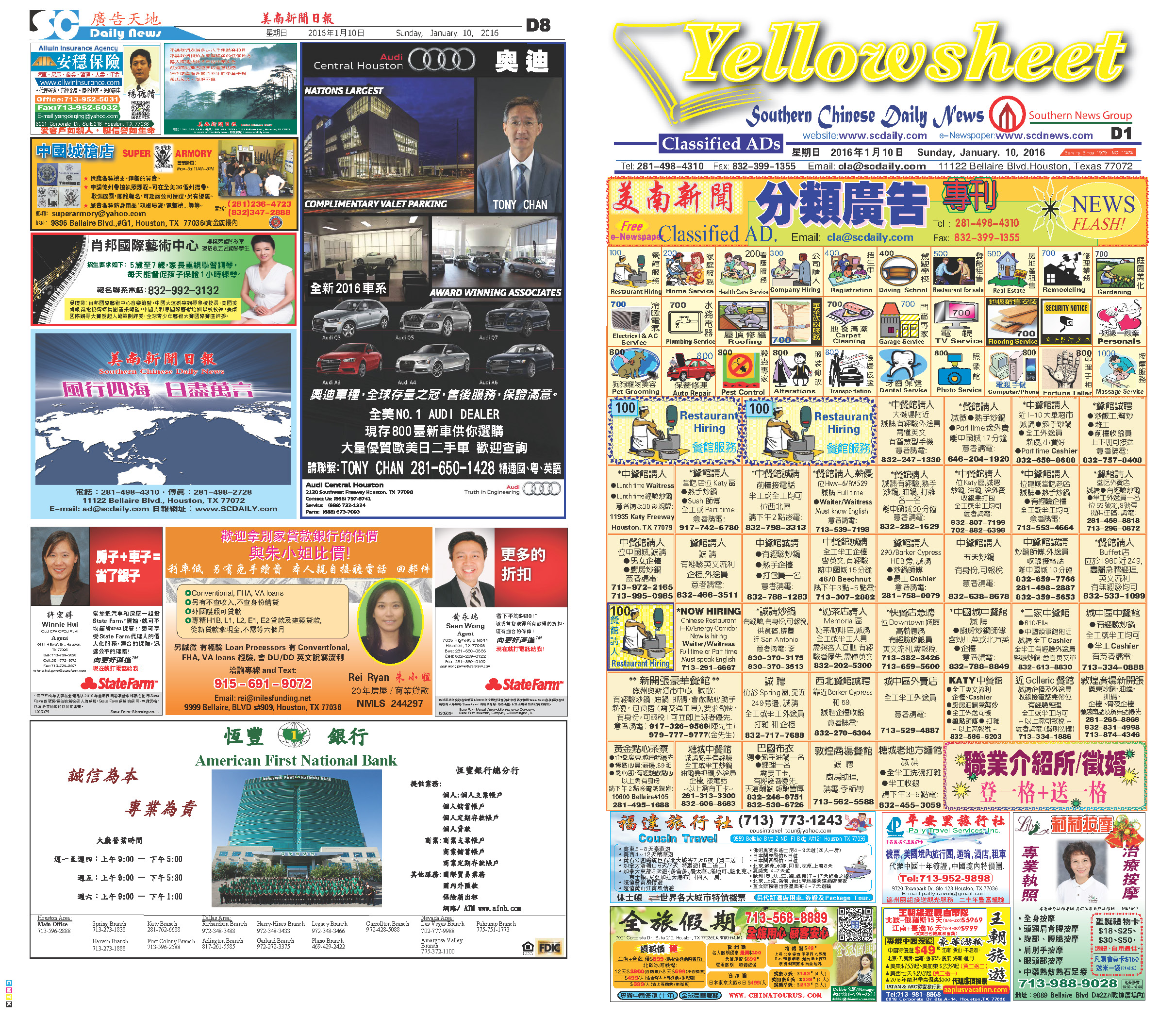160109 Epaper

 A Section B SectionC Section D Section160108 Epaper

 A Section B SectionC Section D Section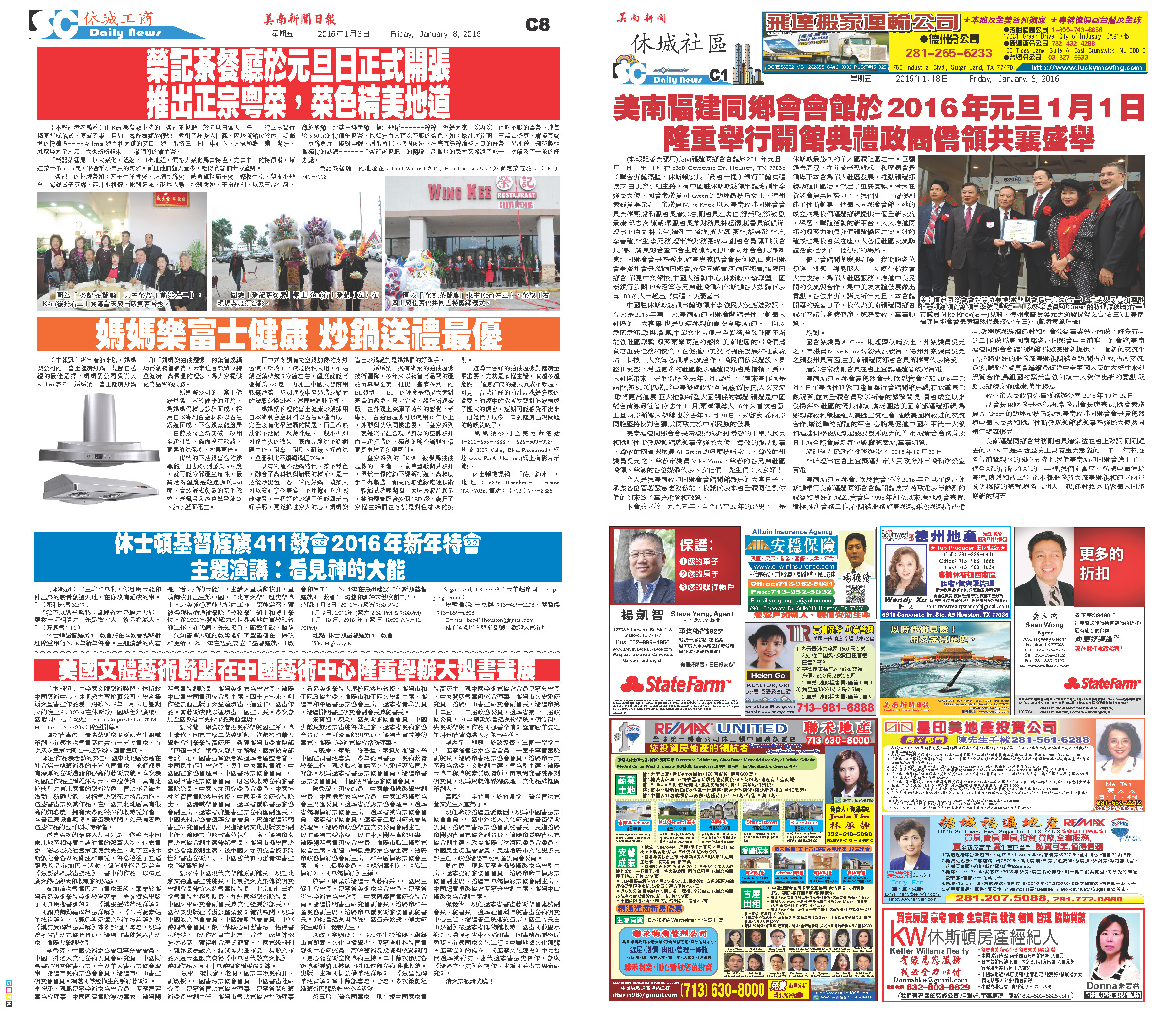160107 Epaper

 A Section B SectionC Section D Section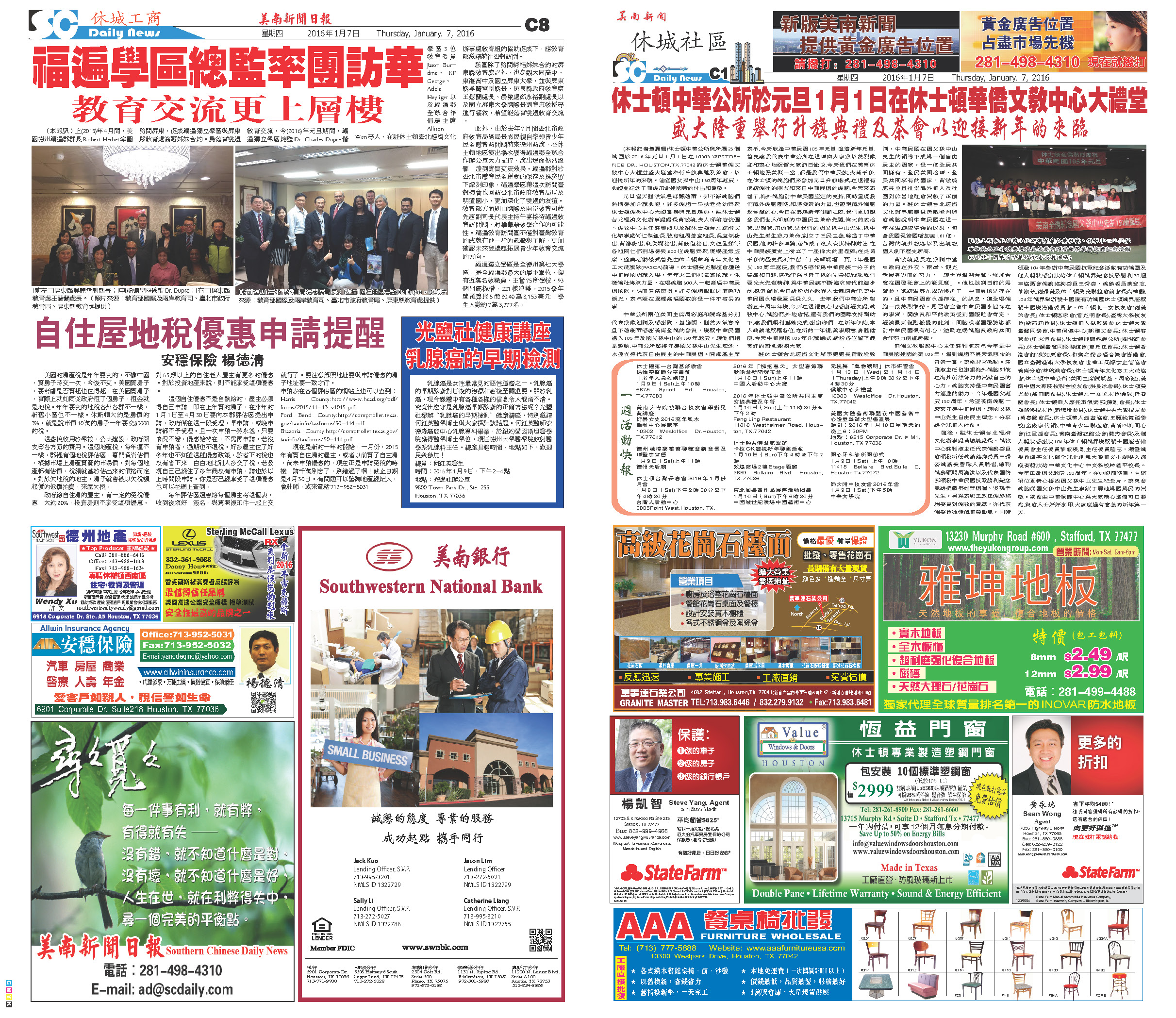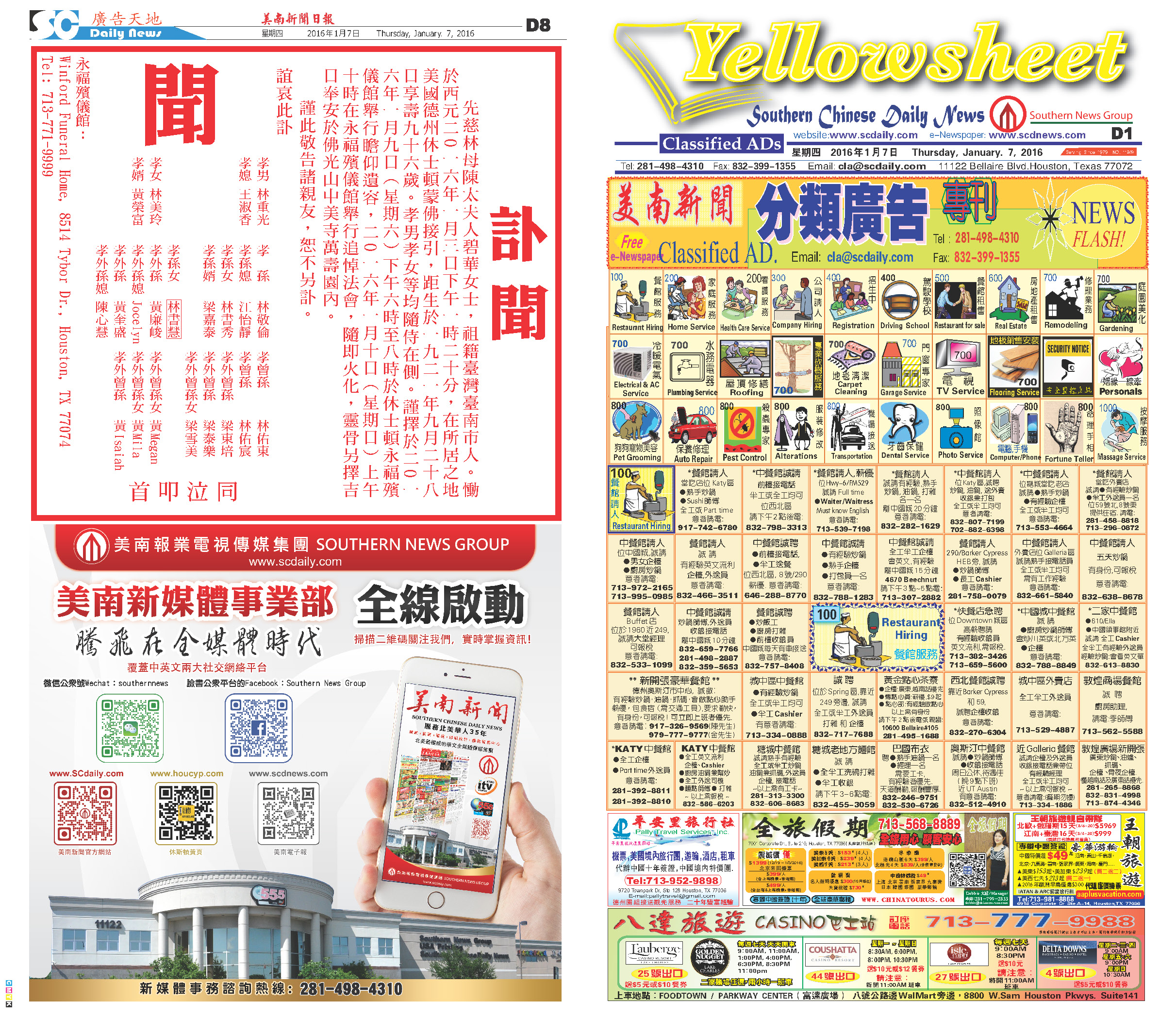160106 Epaper

 A Section B SectionC Section D Section160104 Epaper

 A Section B SectionC Section D Section160105 Epaper

 A Section B SectionC Section D Section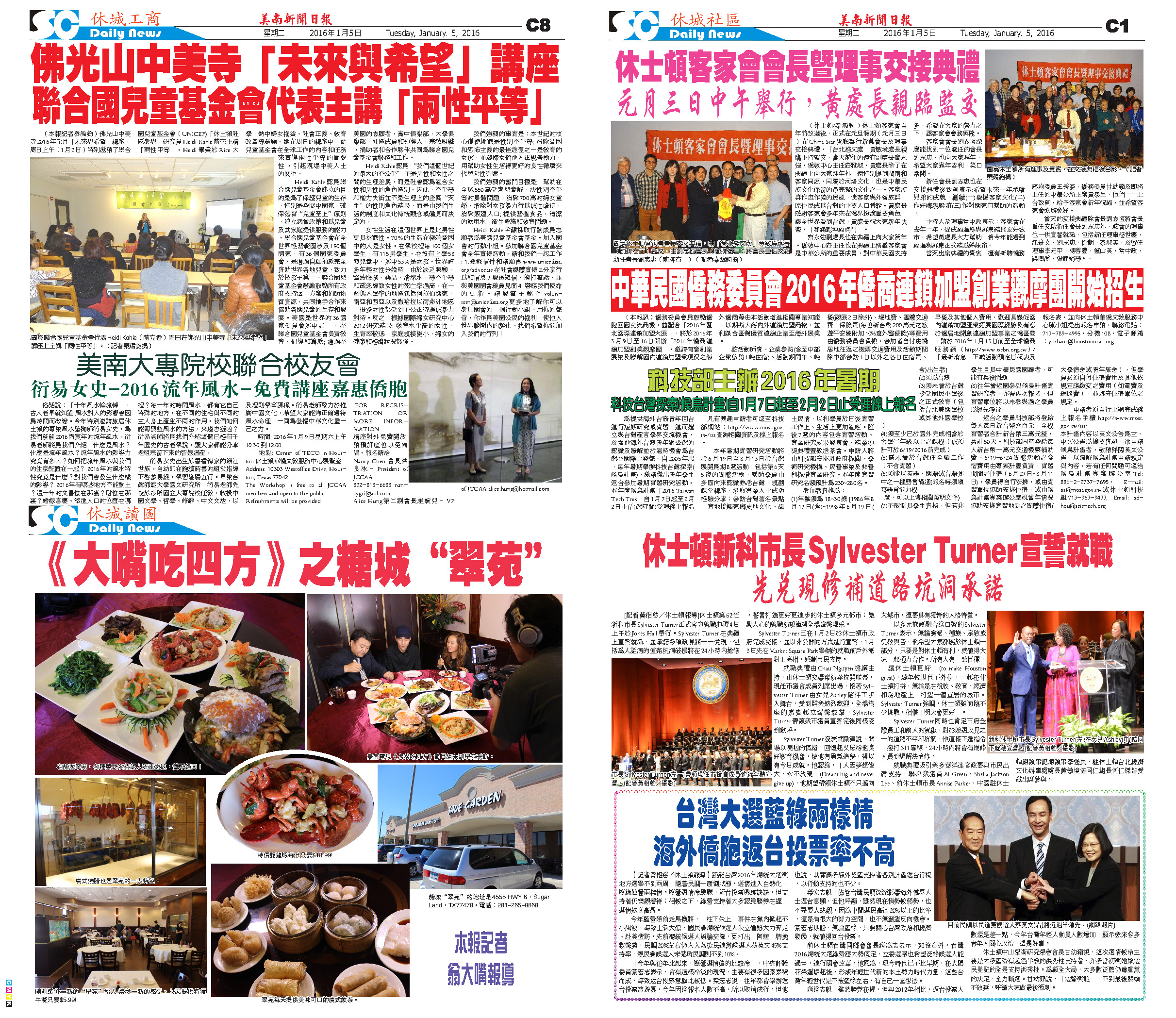160103 Epaper

 A Section B SectionC Section D Section